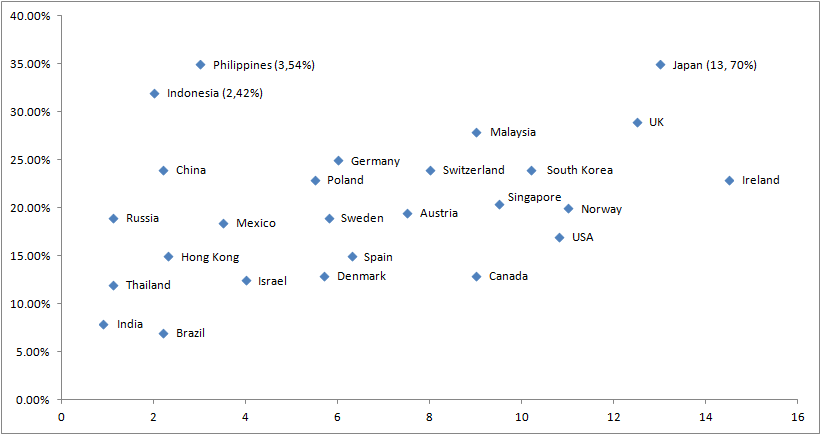### CAT 2008 Question Paper Question 29

Instructions

Directions for the next three questions:

Answer the following questions based on the information given below: Telecom operators get revenue from transfer of data and voice. Average revenue received from transfer of each unit of data is known as ARDT. In the diagram below, the revenue received form data transfer as percentage of total revenue received and the ARDT in US Dollars (USD) are given for various countries.

The X axis represents the ARDT values and the Y axis represents the % of revenue from data transfer as a percentage of the total revenue.Question 29

# It was found that the volume of data transfer in India is the same as that of Singapore. Then which of the following statements is true?

Solution

If the volume of data transfer in India is the same as that of Singapore then we have ($$R_i$$ * 7.5 / 0.9 )= (20*$$R_s$$ / 9.5) ; we get $$R_s$$ nearly equal to 4*$$R_i$$.
Hence  Total revenue in Singapore is about 4 time that of India.